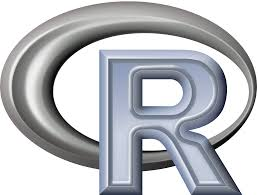####Here is a comprehensive compilation of many R commands that most of the scientists can find useful for analyzing research data.

#### Basics

• Check working directory:
`getwd()`
• Change working directory:
`setwd("path")`
• Show help about a command (quotes are important):
```help("command")
?"command"```
• Show the objects and variables of the working space:
`ls()`
• Remove an object from the working space:
```rm(x)
rm(list=ls()) # Removes all the objects```
• Save the working space:
```save.image() # in the file .RData at the working directory
save(object list,file="myfile.RData") # saves the object list into the chosen file```
`load("myfile.RData")`
• Quit R:
`q()`

#### Vectors and matrices

• Create a vector:
```x=c(1,2,4,8,16)
y=c(1:10)
z=c(rnorm(n))```
• Operations with vectors:
```x=c(x)+n # adds 'n' to each element
z=c(x,y) # combines 2 vectors creating a linear one
z=cbind(x,y) # combines 2 vectors creating a 2 columns matrix
z=rbind(x,y) # combines 2 vectors creating a 2 rows matrix
replace(x,x==0,NA) # Replaces zeros by NA```
• Operations with matrices:
```mat[4,2] # shows the 4th row and the 2ng column
mat[3,] # shows the 3rd row
mat[,2] # shows the 2nd column
mat[,-3] # deletes the 3rd column
mat[-2,] # deletes the 2nd row
mat[1:3,3:5] # show the rows 1 to 3 and the columns 3 a 5```

#### Frames

• Create a frame from a vector list (each vector will be a frame column):
`data.fr=data.frame(x1,x2,x3 …)`
• Create a frame from a matrix:
`as.data.frame(mat)`
• Check if an object is a frame:
`is.data.frame(mat)`
• Convert a frame into a matrix:
`as.matrix(data.frame)`
• Read a tab-separated data file with the names of its columns on the 1st row and save it into a frame:
`data.fr<-read.table("ruta fichero",header=T,sep="t")`
• Show the names of frame columns:
`names(data.fr)`
• Show the names of frame rows:
`row.names(data.fr)`
• Show the number of frame rows:
`nrow(data.fr)`
• Show the first frame rows:
`head(data.fr, n=10) # 10 first rows`
• Create vectors with the name of each column in the frame (and its data)
```attach(data.fr)
detach(data.fr) # remove the vectors```
• Add a row to frame:
`data.fr <- rbind(data.fr,data.frame(colA=1,colB="abc",colC=rnorm(1)))`
• Add a column to a frame:
`data.fr\$colC <- data.fr\$colA + 5 * data.fr\$colB`
• Filter a frame to obtain a subset of data and save it in a new frame:
```subset.frame<-subset(data.fr,colA>=5 & !is.na(colB) | ColC='V')
subset.frame<-subset(data.fr,complete.cases(data.fr)) # Obtain only the rows with data in all the columns```
• Order a frame:
```data.fr[order(data.fr\$colB),] # Order a frame with the values of the column B
data.fr[rev(order(data.fr\$colB)),] # Reverse order```

#### Statistics

• Calculate several statistical parameters from a dataset:
```max(x)
min(x)
mean(x)
median(x)
sum(x)
var(x) # variance covariance matrix
sd(x) # standard deviation
fivenum(x) # Tukey fivenumbers min, lowerhinge, median, upper hinge, max
Table(x) # Matriz de frecuencias
scale(x,scale=T) # centers around the mean and scales by the sd
cumsum(x) # cumulative sum
cumprod(x)
cummax(x)
cummin(x)
rev(x) # reverse the order of values in x```
• Correlation matrix:
`cor(x,y,use="pair") # correlation matrix for pairwise complete data, use="complete" for complete cases`
• Correlation test:
`cor.test(x,y,method=c('pearson'))`
• ANOVA (Analysis of variance):
```aov.1 <- aov(colA~colB,data.fr) # one way analysis of variance (colA contains values and colB contains classes)
aov.2 = aov(colA~colB*colC,data.fr) #do a two way analysis of variance
summary(aov.1) #show the summary table
print(model.tables(aov.1,"means"),digits=3) #report the means and the number of values/class
boxplot(colA~colB,data.fr) #graphical summary appears in graphics window```
• Lineal regresion:
```fit<-lm(colB~colA,data.fr) #basic linear model where x and y can be matrices (see plot.lm for plotting options)
summary(fit)
plot(data.fr\$colA,data.fr\$colB)
abline(fit)```
• Student’s t-Test:
```t.test(x,g)
pairwise.t.test(x,g)
power.anova.test(groups = NULL, n = NULL, between.var = NULL,
within.var = NULL, sig.level = 0.05, power = NULL)
power.t.test(n = NULL, delta = NULL, sd = 1, sig.level = 0.05,
power = NULL, type = c("two.sample", "one.sample", "paired"),
alternative = c("two.sided", "one.sided"),strict = FALSE)```

#### Graphics

• List of available colors in R:
`colors()`
• Graphic parameters:
`help(par)`
• Simple graph::
```plot(x,y,type="p") # types: “p”: points, “l”: line, …
plot(x,y,col="red",lwd=2,type="l")```
• Draw a point:
`points(2,5,pch=16,col="blue",cex=3) # Draws the point (2,5) in blue and with a relative size of 3`
• Label the point:
`text(2,5,label="Point label",pos=4,offset=-0.5,font=3,cex=0.75)`
• Scatter plot:
```plot(data.fr\$colA, data.fr\$colB,
main="Column A vs. Column B", xlab="Column A", ylab="Column B",
cex.main=2, cex.lab=1.5, pch=1)```
• Graph overlay:
```lines(1-spec, sens, type='b', col=39, pch=7, lty=2)
plot(data.fr\$colA, data.fr\$colB,
main="Column A vs. Column C",
• Small graph into a bigger one:
```year <- 1900:2000
dollars <- (year-1899)^2
plot.within.aplot <- function()
{
par(pin=c(1.5,1.5)) ### set the plot region size in inches
par(mai=c(2.8,1.2,1,2.6)) ### set the 4 margin sizes in inches
par(ps=6) ### set the font size to 6 point
plot(year,dollars,col="blue",type="l",xlab="",ylab="") ### no axis labels
par(new=T) ### the next plot will plot over the previous one
par(ps=12) ### set the font size to 12 point
par(pin=c(4.14,3.57)) ### set the plot region size back to the default
par(mai=c(.956,.769,.769,.394)) ### set the margins back to the default
plot(year,dollars,col="green",type="l") ### plot
}
plot.within.aplot()```

#### Data output

• Graph window options:
```X11() # open a new window in Linux
windows() # open a new windows in Windows
dev.list() # show the open windows
dev.cur() # show the working window
dev.off() # close the working window
dev.set(3) # select the window 3 as working one
dev.off(3) # close the window 3
graphics.off() # close all the windows```
• Open a PDF file to output data:
`pdf(file=”outfile.pdf”)`

#### My original article in Spanish:

http://bioinfoperl.blogspot.com/2010/06/algunos-comandos-de-r-utiles-en-ciencia.html